# find % of row value vs. column total

I have a column of various quantities and I want to find the % value of the specific row value when compared to the column total of quantity.  Is this possible in Beast Mode or Dataflow?

• I think I have the exact same question. See screenshot below for question. I'd like a column that would be 325,965 / 959,627  or 34.0% and so on for each subsequent row.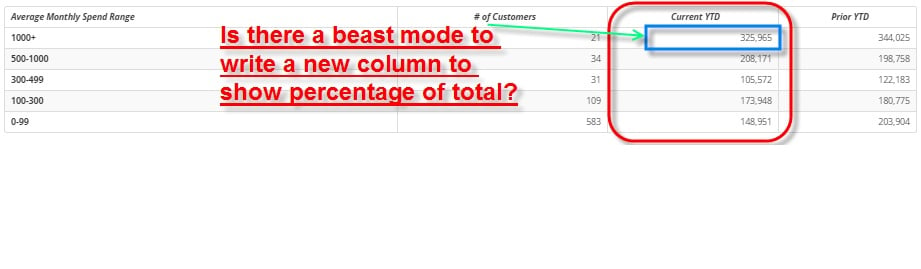• Hi all,

Can anybody help @kivlind and @mwade out?

Thanks!

Sadly in beastmode we cannot calculate this. We will need to use a dataflow to accomplish this.

Using a Magic ETL:
In ETL we first want to replicate our card.

2. If your card has any filters you want to apply them next, using "Filter Rows"
3. We will now use "Add Constants" to add a constant text column of 1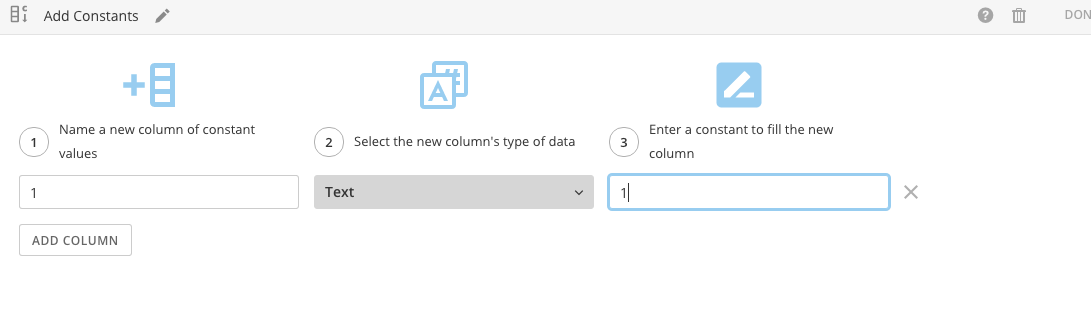4. Now using group by we are going to group by "1" and aggregate our value column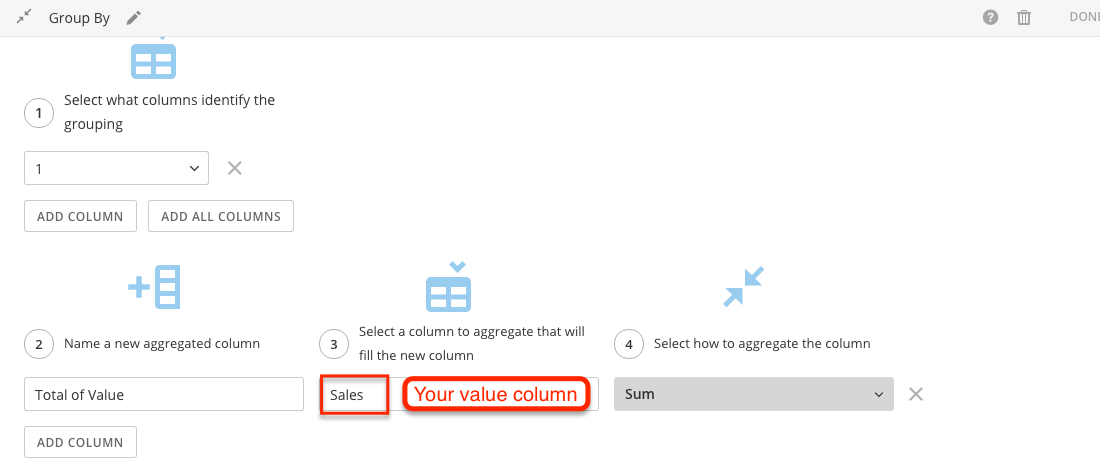1. If you have multiple Value columns you want to aggregate all of them in this step.
5. You should now have a column with a single value in it.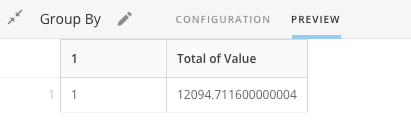1. This is your total for that column
6. You now will want to join this to our original data.From the orignal data we are going to use "Add Constants" and add a constant text column with a value of 1
7. We now can join our input dataset to our single value.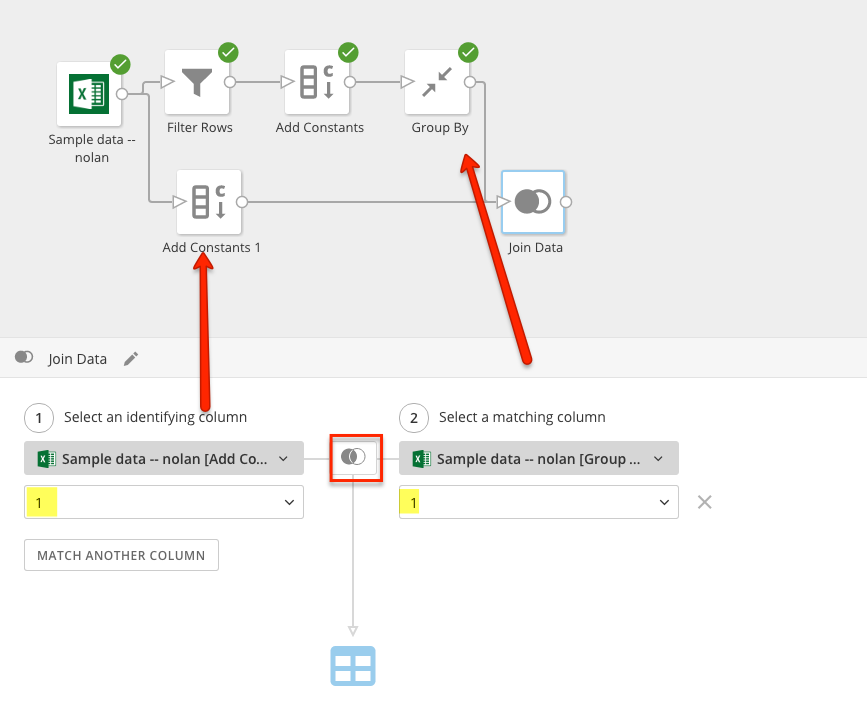8. Add a Select Columns and remove your columns 1 and 1_1

You can now create a card from this Magic ETL. You will now have a column that contains a total value.

You can now create a beastmode to find the percent.

`SUM(`value_column`) / MAX(`total of value`)`

This should give you your percentage.

• Any chance this can get added to the development plan? We need to calculate this % to Total dynamically, based on what the group by is on the card.

• A dynamic solution at card level can be to use a window function. It's not currently documented and may be subject to change, but I've found it to work for basic aggregates: SUM, AVG, MIN, MAX

In your case, you could get the grand total:

SUM(SUM(value)) OVER ()

And for % Total:

SUM(value) / SUM(SUM(value)) OVER ()

• Hi,

Can you explain what OVER() is? I don't think it is found within the Beast mode function list.

Thanks.

• OVER() indicates the function is not a plain aggregation, but an analytic function. It's also called a window function because you can specify a window of rows to evaluate.  In the example above, OVER() is empty which indicates that the SUM is to be across all-time and all groupings, thus returning the grand total.

Here is some documentation for one database vendor...which explains how it generally works.

https://www.vertica.com/docs/9.2.x/HTML/Content/Authoring/AnalyzingData/SQLAnalytics/WindowPartitioning.htm

• What about by count and subtotal?